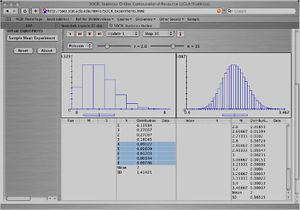# SOCR EduMaterials Activities CLT

## URL Go to: http://socr.umich.edu/html/exp/

### Choose the Sample Mean Experiment. In that experiment, you can show that the Central Limit holds regardless of the distribution of the original random variable if n is large enough

• Central Limit Theorem (CLT): You can read the complete statement, proof and applications of the CLT here!
• Exercise 1: Consider a Poisson random variable X with parameter λ=1.5. Select the Poisson distribution in the applet, and r=1.5 (since r represents the parameter, λ). Then set n=1 to indicate that you have only one random variable. Do not touch anything else. You will see a blue distribution on the right and on the left. The distribution on the left is the theoretical distribution of the X that we specified and the distribution on the right is the theoretical distribution of the sample average (which in the applet is denoted by M). Let's denote the sample average by $$\overline{X}$$!
• Question 1(a): Compare these two theoretical distributions. Attach a snapshot of how they look and the theoretical distribution tables below them. They are exactly the same! Because when n=1, $$\overline{X}$$=X.• Question 1(b): Compare the Mean of the theoretical distribution of the X variable and that of the Mean of the distribution of the (the distribution of the M). These are theoretical means. What is their relation?

The Mean of the theoretical distribution of the X is 1.5, while that of the Mean of the distribution of the is 1.5, too. They are the same. Theoretically, E($$\overline{X}$$) = E(X) is always true if $$\overline{X}$$ is the mean of several independent and identical random variables (IID) no matter how large is n.

• Question 1(c ): Compare the standard deviation of the theoretical distribution of the X variable and the Standard deviation of the distribution of the (the distribution of the M). These are theoretical means. What is their relation? The SD of the theoretical distribution of the X is 1.22474, while that of the SD of the distribution of the sample-average is 1.22474, too. They are the same. Theoretically, SD($$\overline{X}$$) = $$SD(X)\over{\sqrt(n)}$$. Hence they are equal when and only when n=1.

• Question 2: Explain why both distributions in question 1 look like that. They are exactly the same! Because when n=1, $$\overline{X}$$=X.

• Question 3: Let’s consider now 3 random variables X1, X2, X3 independent and identically distributed Poisson random variables, each Poisson with parameter λ=1.5. So, now set r=1.5 and n=3. It is still true, after you do this, that the blue distribution on the left is the theoretical distribution of one Xi and the one on the right is the theoretical distribution of the $$\overline{X}$$. Compare the left and right distributions now. Print a snapshot.

The right distribution is skewed less to the right than the left distribution. As the relationship mentioned above, The Mean of the theoretical distribution of the X is the same as that of the Mean of the theoretical distribution of the . The SD of the theoretical distribution of the X is larger than that of the SD of the theoretical distribution of the $$\overline{X}$$ with relationship SD($$\overline{X}$$)=$$SD(X)\over{\sqrt(3)}$$.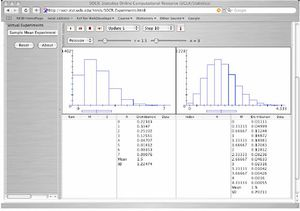• Question 4: Hold the r=5 and the Poisson distribution, but play now with the “n” until you see the Central limit Theorem hold. Print a snapshot of when you think that happens.

How large is the number of random variables you have to use so that their $$\overline{X}$$ is normally distributed? n=8 (the answer may be different!)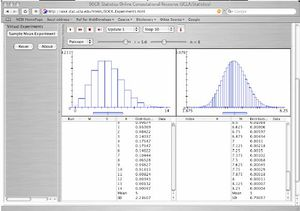• Question 5: The above (question 1-4) were all theoretical. Now we will do the experiment that will lead us to the theoretical result you found in question 4. One run of the experiment would be to get “n” Poisson random numbers (where “n” is the “n” you got in question 4). Let’s do that. Keeping everything as in question 4, just click on the 〉〉 button. You won’t see the actual “n” Poisson random numbers you draw (i.e., you don’t see the but you see their mean “m” on the left hand side, bottom part, and their standard deviation S. You also see how the distribution of the “n” numbers looks. Print a snapshot. How close is your distribution for the “n” draws to the theoretical distribution of the random variable X? How does the mean of the n $$\overline{X}$$’s compare with the theoretical mean of the X? How do the standard deviations compare? Compare them.

The distribution of the 8 numbers (the red distribution in the left side) doesn’t look like the theoretical distribution of X. The mean of the 8 Xi’s is 5, which is the same as the theoretical mean. The SD of the 8 $$\overline{X}$$’s is 2.39045, which is close to the theoretical SD 2.23637.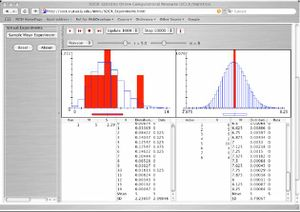• Question 6: Obviously, to obtain the theoretical result on the right hand side that you obtained in question 4 by experimentation requires a lot of repetitions of the experiment you just did in question 5. So let’s repeat the experiment of observing “n” Poisson numbers,” 10,000 times. Put stop=10,000, update=1000 (it may take a little while, so be patient). Print a snapshot of what you get. So does 10,000 repetitions of the experiment get you close to the theoretical distribution of (of M in the applet)? How close? How close is the experimental mean of $$\overline{X}$$ after 10,000 repetitions to the true mean of $$\overline{X}$$?

After 10,000 repetitions, the distribution of the real data mean (the red distribution in the right side) is getting closer to the theoretical distribution of (the blue distribution in the right side). The experiment mean of the distribution is 4.99998, which almost the same as the theoretical mean 5. The experiment SD of the distribution is 0.79296, which is slightly larger the theoretical SD 0.79057.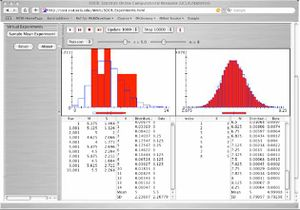• Question 7: This question is to see if you really understood what you did above. The number of accidents per week at a cross road is a Poisson random variable with parameter λ=2.
• How many weeks would we need to observe the cross-road until the observed average number of accidents in all weeks is normally distributed? Use the SOCR applet to answer this question. Print a snapshot that illustrates the answer you write here.

When n=15, the distribution of $$\overline{X}$$ looks like the normal distribution. (The answer may be different). Hence we need to observe 15 weeks until the observed average number of accidents in all weeks is normal distributed.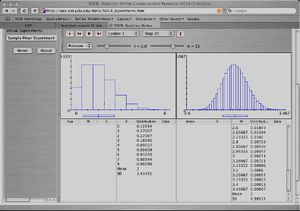• According to your result in (a), what is the approximate probability that the average number of accidents per week is bigger than 3? Compute the exact probability, too. Justify your answer for both questions. Highlight your answer in the snapshot.

Set stop = 10,000 and update = 1,000, the data show that the approximate probability that the average of accidents per week is bigger than 3 is: P($$\overline{X}$$ >3) = the summation of the highlight numbers in “Data” column = 0.0032.

The exact probability can be computed from the theoretical distribution of $$\overline{X}$$, that is: P($$\overline{X}$$ >3) = the summation of the highlight numbers in “Distribution” column = 0.00386.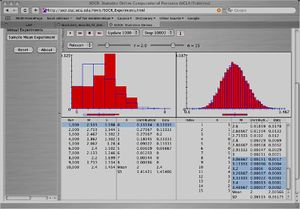• What is the probability of seeing more than 3 accidents in one week? Highlight your answer in the snapshot. P(X>3) = summation of the highlight numbers = 0.14264.